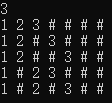• n个节点二叉树有多少种形态（Catalan数）】 分析过程： （1）先考虑只有一个节点的情形，设此时的形态有f(1)种，那么很明显f(1)=1 （2）如果有两个节点呢？我们很自然想到，应该在f(1)的基础上考虑递推关系。...
【n个节点的二叉树有多少种形态（Catalan数）】
分析过程：
（1）先考虑只有一个节点的情形，设此时的形态有f(1)种，那么很明显f(1)=1
（2）如果有两个节点呢？我们很自然想到，应该在f(1)的基础上考虑递推关系。那么，如果固定一个节点后，左右子树的分布情况为1=1+0=0+1，故有f(2) = f(1) + f(1)
（3）如果有三个节点，（我们需要考虑固定两个节点的情况么？当然不，因为当节点数量大于等于2时，无论你如何固定，其形态必然有多种）我们考虑固定一个节点，即根节点。好的，按照这个思路，还剩2个节点，那么左右子树的分布情况为2=2+0=1+1=0+2。
所以有3个节点时，递归形式为f(3)=f(2) + f(1)*f(1) + f(2)。(注意这里的乘法，因为左右子树一起组成整棵树，根据排列组合里面的乘法原理即可得出)
（4）那么有n个节点呢？我们固定一个节点，那么左右子树的分布情况为n-1=n-1 + 0 = n-2 + 1 = … = 1 + n-2 = 0 + n-1。此时递归表达式为f(n) = f(n-1) + f(n-2)f(1) + f(n-3)f(2) + … + f(1)f(n-2) + f(n-1)
接下来我们定义没有节点的情况，此时也只有一种情况，即f(0)=1
那么则有:
f(0)=1，f(1)=1
f(2)=f(1)f(0)+f(0)f(1)
f(3)=f(2)f(0)+f(1)f(1)+f(0)f(2)
.
.
.
.
f(n)=f(n-1)f(0)+f(n-2)f(1)+……….+f(1)f(n-2)+f(0)f(n-1)
该数列称为卡特兰数（Catalan数），该递推关系的解为：
这里写图片描述
即含n个节点的二叉树有f(n)种形态。
【其他使用Catalan数解决的问题】
(1)矩阵链乘： P=a1×a2×a3×……×an，依据乘法结合律，不改变其顺序，只用括号表示成对的乘积，试问有几种括号化的方案？
(2)一个栈(无穷大)的进栈序列为1,2,3,…n,有多少个不同的出栈序列?
(3)有2n个人排成一行进入剧场。入场费5元。其中只有n个人有一张5元钞票，另外n人只有10元钞票，剧院无其它钞票，问有多少中方法使得只要有10元的人买票，售票处就有5元的钞票找零？(将持5元者到达视作将5元入栈，持10元者到达视作使栈中某5元出栈)
(4)将一个凸多边形区域分成三角形区域的方法数?
(5)在圆上选择2n个点,将这些点成对连接起来，使得所得到的n条线段不相交的方法数。
(6)一位大城市的律师在她住所以北n个街区和以东n个街区处工作。每天她走2n个街区去上班。如果她从不穿越（但可以碰到）从家到办公室的对角线，那么有多少条可能的道路？


展开全文• 如题，考研数据结构问题，具有n个结点且深度也为n的二叉树有多少种？数据结构
• 1个节点二叉树只有1种形态，A=1 2）n个节点n>=2）的二叉树有 A[n] = ∑ [m=0到n-1] ( A[m]*A[n-m-1] ) ，求和的每一项，分别表示根的左子树为m个节点、右子树为 n-m-1个节点的情况 刚好就是catalan数，直接用...
记n个节点的二叉树形态个数为A[n]
1）0个节点的二叉树只有1种形态，A=0；1个节点的二叉树只有1种形态，A=1
2）n个节点（n>=2）的二叉树有 A[n] = ∑ [m=0到n-1] ( A[m]*A[n-m-1] ) ，求和的每一项，分别表示根的左子树为m个节点、右子树为 n-m-1个节点的情况 刚好就是catalan数，直接用catalan数的公式：h(n)=C(2n,n)/(n+1)
http://zhidao.baidu.com/question/407856789.html?qbl=relate_question_0&word=5%B8%F6%BD%DA%B5%E3%20%B6%FE%B2%E6%CA%F7

展开全文• 具有3个结点二叉树有几种形态？ 正确答案: B 你的答案: B (正确) 4 5 6 7 解析 这是一道牛客网上的测试题，因为题目是求3个节点二叉树的形态，所以直接手画了。...


具有3个结点的二叉树有几种形态？

正确答案: B   你的答案: B (正确)

4

5

6

7

解析
这是一道牛客网上的测试题，因为题目是求3个节点的二叉树的形态，所以直接手画了。但是看解析的时候，发现评论区居然有计算公式，真的是大开眼界啊。

那个回答者给出的答案是：

这是组合计数问题，最常见的catalan数，C(n)=(1/(n+1))*((2*n)!/(n!*n!))

C(3) = (2*3)!/(3！*3！)/(3+1)=5

链接：具有三个节点的二叉树有多少种形态的解析


展开全文• 我们知道具有n个结点的不同的二叉树的数量是(这是一卡塔兰数)，那么如何确定这些二叉树是什么样子的呢？下面是博主的思路： 我们还知道一入栈序列的不同出栈序列的数量也是，于是博主就想：这不是巧了么，...
        我们知道具有n个结点的不同的二叉树的数量是个(这是一个卡塔兰数)，那么如何确定这些二叉树是什么样子的呢？下面是博主的思路：

我们还知道一个入栈序列的不同出栈序列的数量也是个，于是博主就想：这不是巧了么，既然他们的数量是一样的，而且均不相同，那么是不是可以构建一个映射，使得不同的出栈序列和不同的二叉树进行一一对应呢。

于是，在博主的做了深入的观察和思考后，发现对于其中一个出栈序列，其实和某个二叉树的中序遍历结果是相对应的，而经过进一步探索，发现入栈序列是所有二叉树的前序遍历结果。

众所周知，一个二叉树的前序和中序遍历结果是可以唯一确定一棵二叉树的。

然而在写代码的过程中博主又遇到了一个难题：就是如何输出一个入栈序列的全部出栈序列呢？为了解决这个问题，博主上某搜索网站参考了各位大神的代码，发现他们基本都是用回溯法解决的。于是在一番研究之下。我成功地写出了输出一个入栈序列的全部出栈序列的函数。

这样一来，所有问题都解决了。然后经过了20分钟的编码。博主成功地写出了求解具有n个结点的不同的二叉树的所有前序增强序列的C++代码：

另外如果大家有什么疑问或者见解可以在评论下方讨论，如果发现确实有什么错误的话，还请指正。

如下所示：

#include <iostream>

using namespace std;

static const int M = 100;
//依次代表的意思是结点数、栈、出栈序列、栈指针、出栈序列大小、输入顺序、二叉树中序序列，二叉树中序序列索引
static int n,stk[M],seq[M],stk_top=-1,seq_size=0,input[M],in[M],idx;

//根据二叉树的前序和中序遍历获取增强前序序列
static void getEnhencedPreorder(int root, int begin, int end) {
if (begin > end) {
cout << "#" << " ";
return;
}
int i = begin;
while (input[root] != in[i]) {
i++;
}
cout << input[root] << " ";
getEnhencedPreorder(root + 1, begin, i - 1);
getEnhencedPreorder(root + (i - begin) + 1, i + 1, end);
}

//获取所有出栈序列
static void getSequence(int i) {
if (i == n) {
//收集结果
idx = 0;
for (int i = 0; i < seq_size; i++) {
in[idx++]=seq[i];
}
for (int i = stk_top; i >= 0; i--) {
in[idx++] = stk[i];
}
//根据前序和中序遍历获取其增强前序遍历序列
getEnhencedPreorder(0,0,n-1);
cout << endl;
} else {
//对于一个入栈的元素，可以入栈，也可以不入栈
//但是每种情况都要检验

//一、首先是元素入栈了
stk[++stk_top]= input[i];
getSequence(i + 1);
stk_top--;

//二、然后元素没有入栈，并且栈顶元素出栈了
if (stk_top!=-1) {
int top= stk[stk_top--];
seq[seq_size++]=top;
getSequence(i);
seq_size--;
stk[++stk_top] = top;
}
}
}

//相当于main函数
void stack_pop_sequence_re2_main() {
cin >> n;
for (int i = 0; i < n;i++) {
input[i] = i + 1;
}
getSequence(0);
}

下面是一个示例(当n取3时)：

其入栈序列为1 2 3，输出结果为所有组成的二叉树的前序增强序列('#'号标识空)。展开全文c++ 算法 数据结构 c语言
• n个无差别的节点构成的二叉树有多少种不同的结构？给定一整数n，请返回不同结构的二叉树的个数。保证结果在int范围内。测试样例：1返回：1class TreeCount {public: int Cmn(int m,int n) { if(m==n||n==0) ...
• 线索二叉树中为什么n个结点二叉树中，有n+1和空指针： 因为每结点有2空指针，而每度为1的结点有1空指针， 则总的空指针数为 2 * n0 + n1 而 n0 = n2 + 1 (二叉树的特性) 所以对上式化简，德总的空...数据结构
•数据结构
• n个节点二叉树形态数为A[n]1）0个节点二叉树只有1种形态，A=0；1个节点二叉树只有1种形态，A=12）n个节点n>=2）的二叉树有 A[n] = ∑ [m=0到n-1] ( A[m]*A[n-m-1] ) ，求和的每一项，分别表示...
• 1 、计算N节点能够组成的二叉树个数 可以分析，当n=1时，只有1个根节点，则只能组成1种形态的二叉树，令n节点可组成的二叉树数量表示为h(n)，则h(1)=1; h(0)=1; 当n=2时，1个根节点固定，还有2-1个节点。这一...数据结构
• 问题：具有3个结点二叉树有几种形态？ A.4 B.5 C.6 D.7 解析：正确答案: B 你的答案: B(正确)。 C(n)=(1/(n+1))*((2*n)!/(n!*n!)) C(3) = (2*3)!/(3！*3！)/(3+1)=5 【n个节点二叉树有多少种形态...算法
• 输出n个节点二叉树有多少种形态 或 二、实现 import java.util.HashMap; public class Test{ public static void main(String args[]) { int n = 4; int res = getRootNum(n); System.out....
• 1.n个节点二叉树，最多可以有多少层？ A.n/2 B.log(n) C.n-1 D.n 答案解析： D 假设从根节点开始，根节点的层数为1，每一层一个节点，则有n层。数据结构 java
• ## n个节点的二叉树有多少种形态

万次阅读 多人点赞 2015-06-28 17:32:49
n个节点二叉树有多少种形态（Catalan数）】分析过程： （1）先考虑只有一个节点的情形，设此时的形态有f(1)种，那么很明显f(1)=1（2）如果有两个节点呢？我们很自然想到，应该在f(1)的基础上考虑递推关系。那么...卡特兰数
• 具有1102个结点的完全二叉树一定有__叶子结点。 79 551 √ 1063 不确定 设n2为度为bai2的节点，n1为度为1的节点，dun0为度为0的节点； 边数n=节点zhi数-1，即n=1101； n=2n2+n1; 完全二dao叉树度为1的节点只能...
• n个节点二叉树的数目： f(0) = f(1) = 1; f(n) = f(n-1)*f(0) + f(n-2)*f(1)+ ... + f(n-i)*f(i-1)+... + f(0)*f(n-1) ; 这是 卡特兰数（ Catalan numbc
• 高度为n的平衡二叉树最少需要多少个节点？高度为n的平衡二叉树最少需要多少个节点？参考 高度为n的平衡二叉树最少需要多少个节点？ 设f（n）为高度为n的平衡二叉树最少含有的节点数，则：f(1) = 1;f(2) = 2; f(3) = ...
• 题目： 利用归纳法证明：一n个结点的非空二叉树的高度至少为 证明： ...将n个结点的非空二叉树分成左子树k个结点和右子树n-k-1个结点，于是结点数为n的树高度 由假设可知： 当时，即时， 故证。
• N个节点二叉树有多少种形态   这是一道阿里的面试题。其实算不上新鲜，但是我之前没关注过，如今碰到了，就顺便探讨下这问题吧：） 拿到这题，首先想到的是直接写出表达式肯定不行，所以有必要从递推入手。...
• 二叉树具有以下五性质：1. 在二叉树的第ｉ(ｉ>=１)层最多有２＾(ｉ - １)个结点。...4. 具有n个结点的完全二叉树的深度为int_UP(log(2，ｎ+1))。5. 如果将一棵有n个结点的完全二叉树自顶向下，同...
• 具有n个结点的不同形态的树的数目 Bn=An-1 ,应该和具有n-1个结点的不同二叉树的数目相同。 具有n个结点的不同形态的森林树木 Dn=An。 【ps: C(n 2n) 为组合，上面为n，下面为2n 】 转自数据结构与算法C++版c语言
• 没耐心的同学们可以直接拉到最底下看结论，有兴趣的话可以浏览全篇文章 ...一棵完全二叉树具有1000个结点，则此完全二叉树有多少度为2的结点？ 完全二叉树699个节点，则叶子节点有多少？ 已知完全二叉树...
• 考虑1叶子节点和0叶子节点的极端情况： 故：...